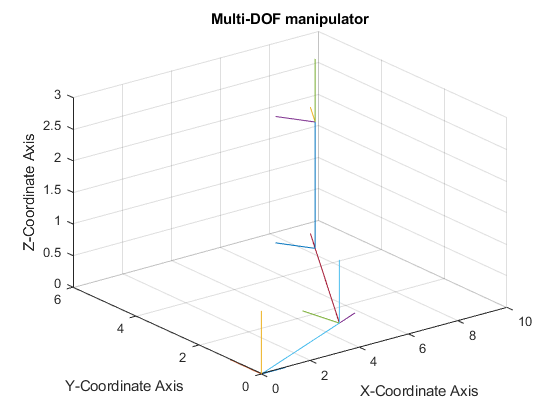Author: Yash Bansod
GitHub: https://github.com/YashBansod

This is the main program. Just call DH_main in command line or run this file to run the program.

## Clear the environment and the command line

``````clear;
clc;
close all;
``````

## Add the directory containing relevant functions to the path variables

``````addpath('./DH-functions/')
``````

## Define the DH table parameters

``````a = [5, 5, 0];                      % Link Lengths (Along X axis)
alpha = [0, 0, 0];                  % Link Twist (Across X axis)
d = [0, 0, 2];                      % Link Offset (Along Z axis)
theta = [15, 30, 0];                % Link Rotation (Across Z axis)
``````

## Call the function that creates and plots the Manipulator

``````DH_table(alpha, a, d, theta);
``````

## Results